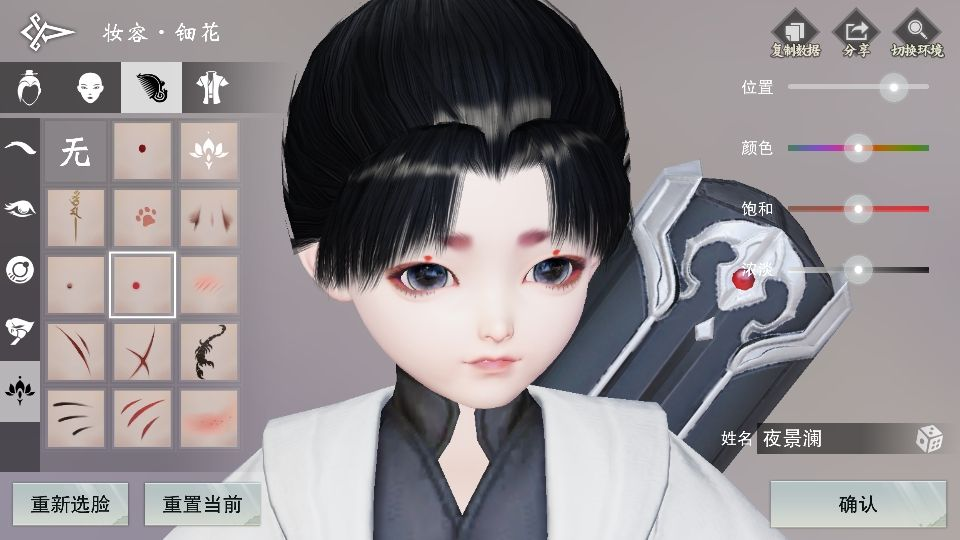Face-to-ParameterTranslationforGameCharacterAuto-Creation

## Dataset

Dimension 2D
Size 512
Format R8G8B8A8_UNORM
Depth Buffer At least 16 bits depth (no stencel)
Enable Mipmap False
Wrap Mode Clamp
Filter Mode Bilinear
Aniso Level 0

Texture2D tex = new Texture2D(rt.width, rt.height, TextureFormat.RGBA32, false);
tex.ReadPixels(new Rect(0, 0, rt.width, rt.height), 0, 0);
tex.Apply();
byte[] bytes = tex.EncodeToJPG();
try
{
File.WriteAllBytes(EXPORT + name + ".jpg", bytes);
}
catch (IOException ex)
{
Debug.Log("转换图片失败" + ex.Message);
}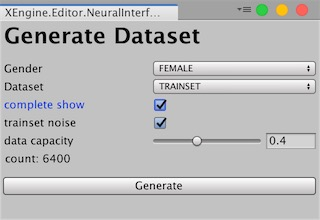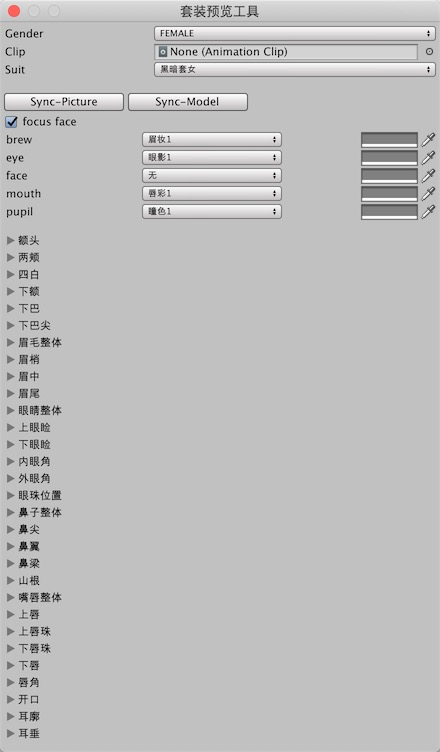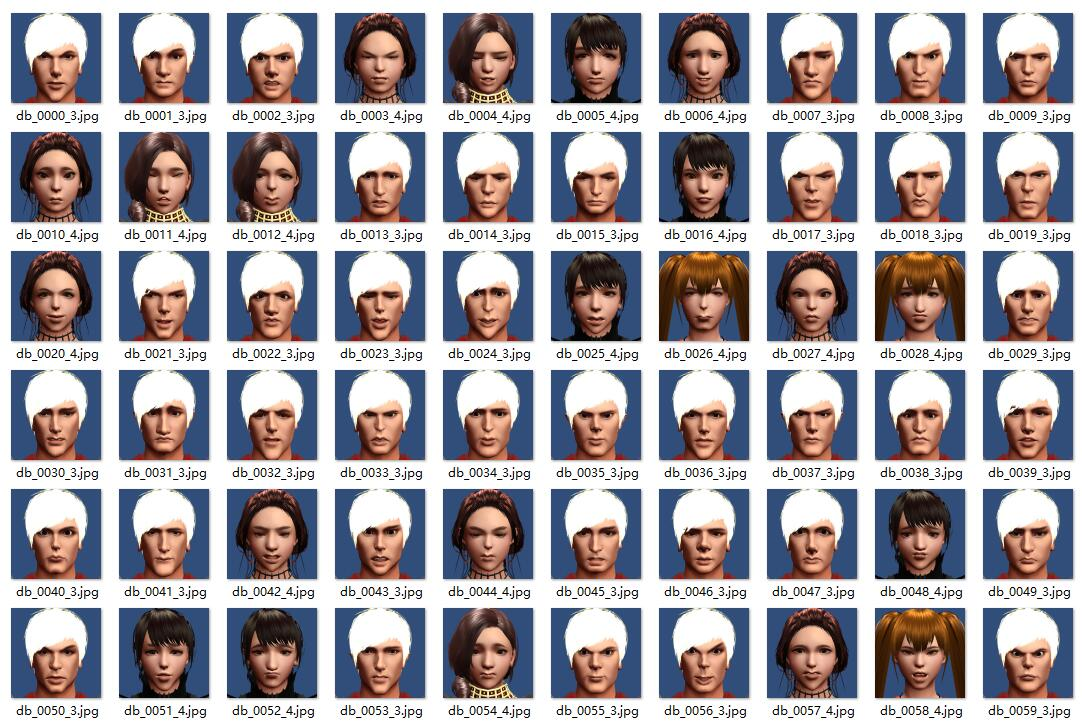## 网络模型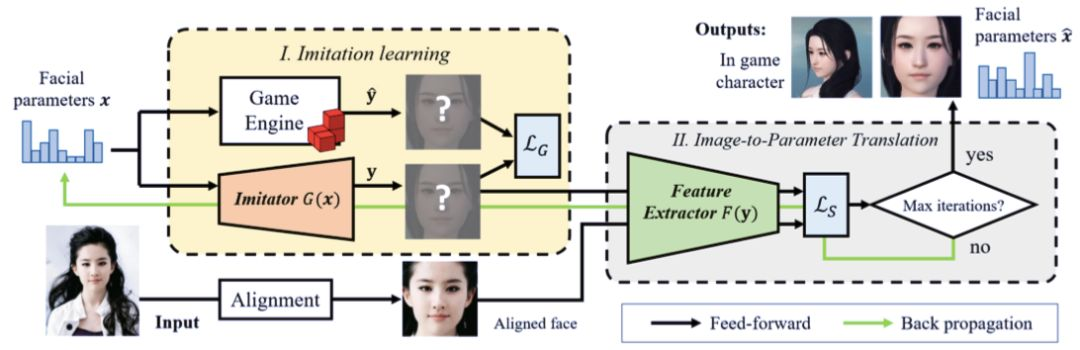### Imatator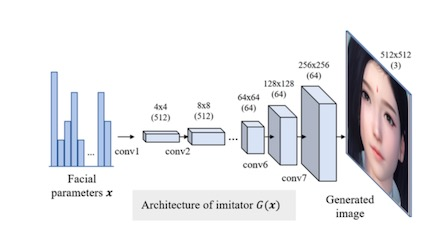def deconv_layer(self, in_chanel, out_chanel, kernel_size, stride=1, pad=0):
return nn.Sequential(nn.ConvTranspose2d(in_chanel,
out_chanel,
kernel_size=kernel_size,
stride=stride,
padding=pad),
nn.BatchNorm2d(out_chanel),
nn.ReLU())

self.model = nn.Sequential(
deconv_layer(args.params_cnt, 512, kernel_size=4),
deconv_layer(512, 512, kernel_size=4, stride=2, pad=1),
deconv_layer(512, 512, kernel_size=4, stride=2, pad=1),
deconv_layer(512, 256, kernel_size=4, stride=2, pad=1),
deconv_layer(256, 128, kernel_size=4, stride=2, pad=1),
deconv_layer(128, 64, kernel_size=4, stride=2, pad=1),
deconv_layer(64, 64, kernel_size=4, stride=2, pad=1),
nn.ConvTranspose2d(64, 3, kernel_size=4, stride=2, padding=1),
nn.Sigmoid(),
)


x = step / float(total_steps)
lr = self.args.learning_rate * (x ** 2 - 2 * x + 1) + 2e-3


### Facial Similarity Measurement 面部相似测量

#### Discriminative Loss 判别损失

，两个向量间的余弦距离可以表示为：

l1 = torch.cosine_similarity(x1, x2)


#### Facial Content Loss 面部内容损失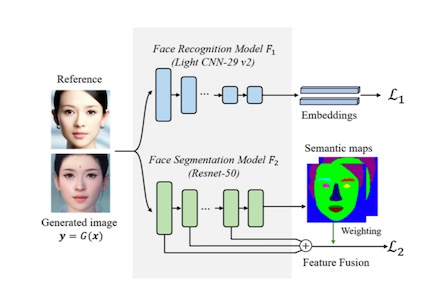# [eyebrow，eye，nose，teeth，up lip，lower lip]
w_r = [1.1, 1.1, 1., 0.7, 1., 1.]
w_g = [1.1, 1.1, 1., 0.7, 1., 1.]
part1, _ = faceparsing_tensor(self.l2_y, self.parsing, w_r, cuda=self.cuda)
y_ = y_.transpose(2, 3)
part2, _ = faceparsing_tensor(y_, self.parsing, w_g, cuda=self.cuda)
loss2 = F.l1_loss(part1, part2)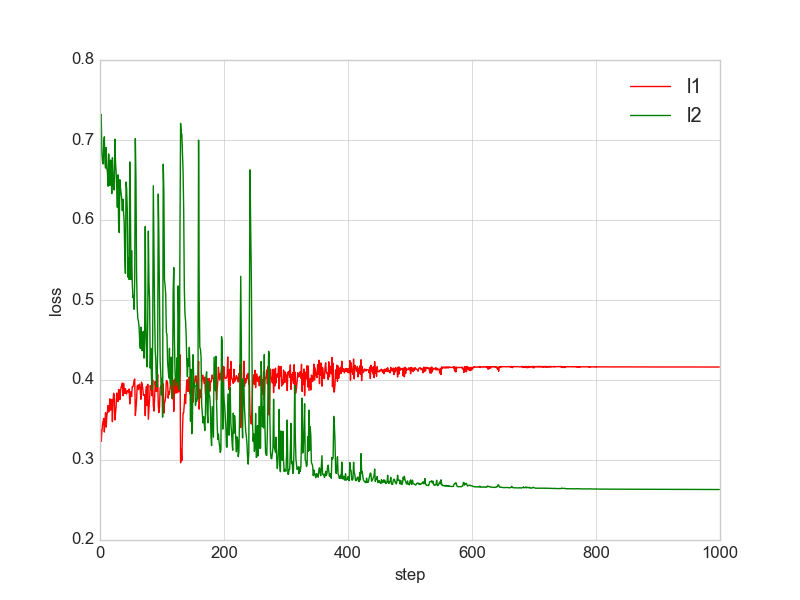（上图红色曲线是余弦相似度的变化， 实际l1=1-cos）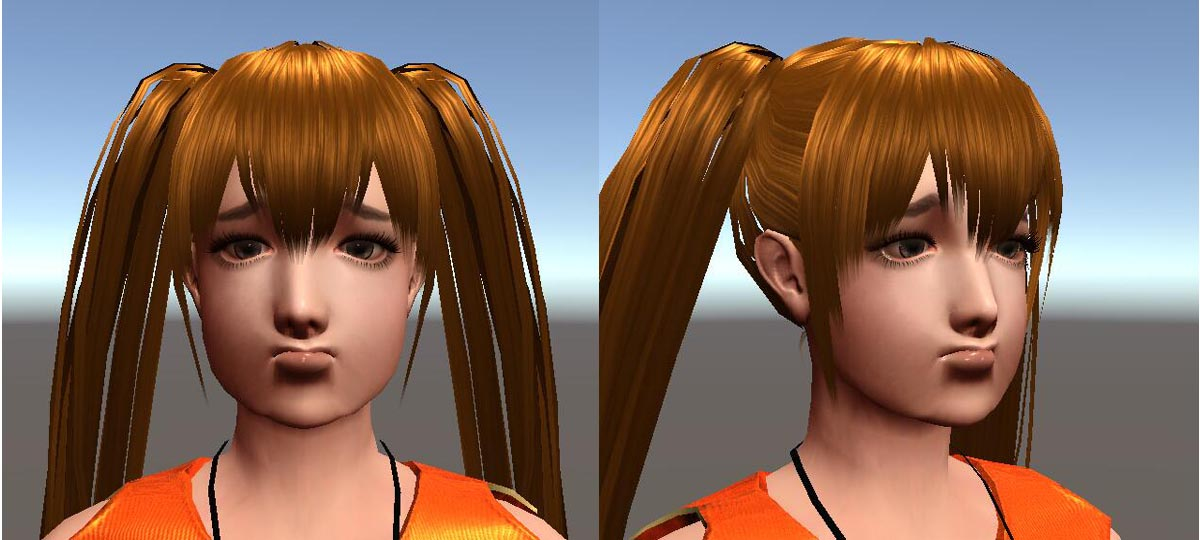### 写在最后：

• 对于外部的图片，一般我们建议首先使用dlib截面部的图， 往往dlib生成的图片和trainset的图片脸部整体轮廓存在一个的偏差， 我们建议先去PS里微调一下， 比如说dataset没有脖子，而dlib却保留了较多部分的脖子。因为我们脸部语义分割网络定义的loss 函数是了l1, 稍微调整之后，能大大加快网络的收敛速度。

• 可能读者更希望看到我们的神经网络能够还原的不仅仅是testset脸部的参数, 而是像论文里还原出一些明星的脸型，甚至是自己上传的一些照片的脸型，这当然是可行的。但这对游戏里的模型提出了一定要求，游戏里拉扯骨骼不能带来畸变或者穿帮， 即蒙皮的时候需更符合物理， 我在调试测试的模型的时候，稍微拉扯眉毛的骨骼，眼珠或者发型就穿帮了， 所以控制这些参数的范围其实设置了一个很小的范围值。